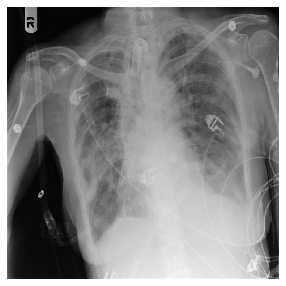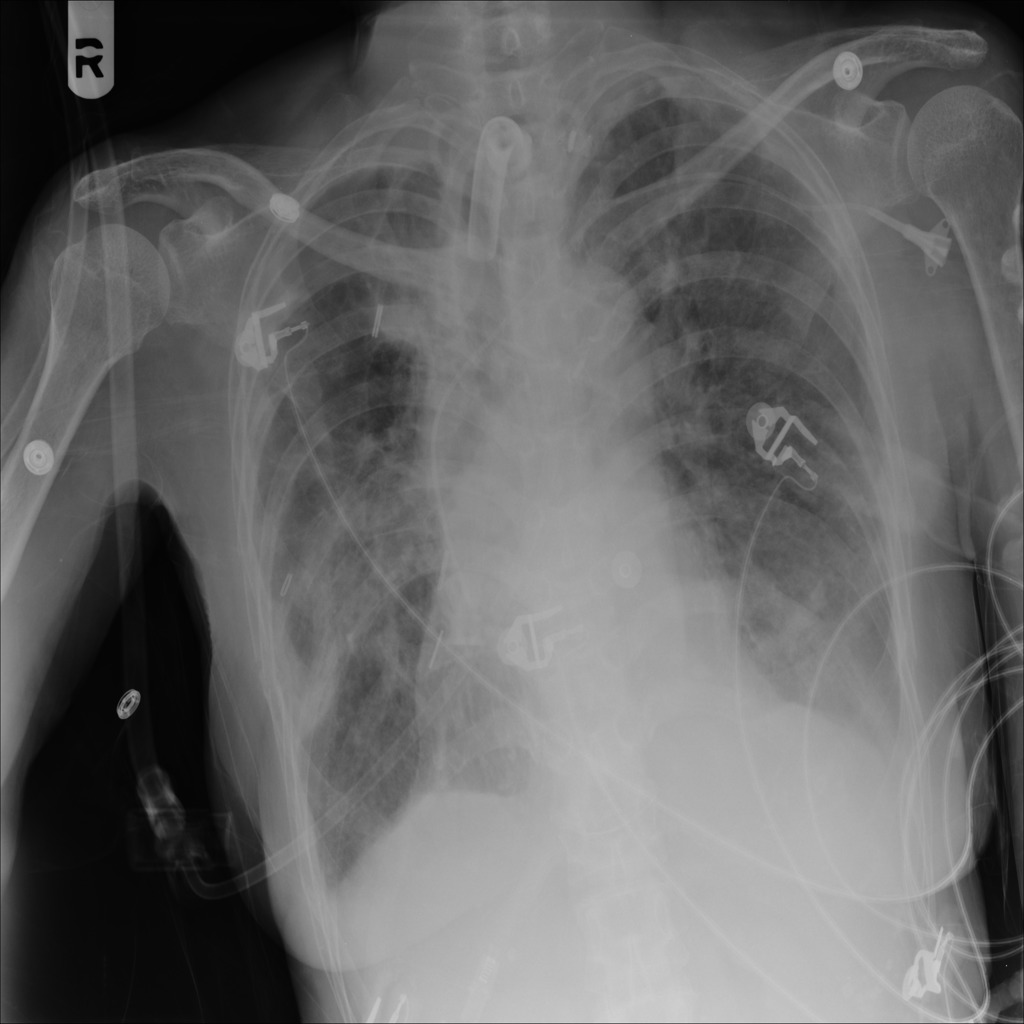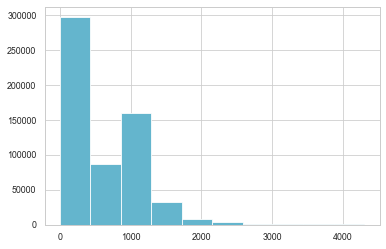Note: This is based on notebook 60_medical_imaging which can be found here

Import the libraries

from fastai.basics import *
from fastai.vision.all import *
from fastai.data.transforms import *
from fastai.medical.imaging import *

import pydicom,kornia,skimage

try:
import cv2
except: pass

import seaborn as sns
sns.set(style="whitegrid")
sns.set_context("paper")

#Load the Data
pneumothorax_source = untar_data(URLs.SIIM_SMALL)


### Patching

##### get_dicom_files

Provides a convenient way of recursively loading .dcm images from a folder. By default the folders option is set to False but you could specify a specific folder if required

#get dicom files
items = get_dicom_files(pneumothorax_source, recurse=True, folders='train')
items

(#250) [Path('C:/Users/avird/.fastai/data/siim_small/train/No Pneumothorax/000000.dcm'),Path('C:/Users/avird/.fastai/data/siim_small/train/No Pneumothorax/000002.dcm'),Path('C:/Users/avird/.fastai/data/siim_small/train/No Pneumothorax/000005.dcm'),Path('C:/Users/avird/.fastai/data/siim_small/train/No Pneumothorax/000006.dcm'),Path('C:/Users/avird/.fastai/data/siim_small/train/No Pneumothorax/000007.dcm'),Path('C:/Users/avird/.fastai/data/siim_small/train/No Pneumothorax/000008.dcm'),Path('C:/Users/avird/.fastai/data/siim_small/train/No Pneumothorax/000009.dcm'),Path('C:/Users/avird/.fastai/data/siim_small/train/No Pneumothorax/000011.dcm'),Path('C:/Users/avird/.fastai/data/siim_small/train/No Pneumothorax/000012.dcm'),Path('C:/Users/avird/.fastai/data/siim_small/train/No Pneumothorax/000014.dcm')...]

Pydicom is a python package for parsing DICOM files and makes it easy to covert DICOM files into pythonic structures for easier manipulation. Files are opened using pydicom.dcmread

img = items
type(dimg)

pydicom.dataset.FileDataset

#### Understanding Dicoms

Tip: This is a quick overview of understanding dicoms

You can now view all the information within the DICOM file. Explanation of each element is beyond the scope of this tutorial but this site has some excellent information about each of the entries. Information is listed by the DICOM tag (eg: 0008, 0005) or DICOM keyword (eg: Specific Character Set)

dimg

(0008, 0005) Specific Character Set              CS: 'ISO_IR 100'
(0008, 0016) SOP Class UID                       UI: Secondary Capture Image Storage
(0008, 0018) SOP Instance UID                    UI: 1.2.276.0.7230010.3.1.4.8323329.6340.1517875197.696624
(0008, 0020) Study Date                          DA: '19010101'
(0008, 0030) Study Time                          TM: '000000.00'
(0008, 0050) Accession Number                    SH: ''
(0008, 0060) Modality                            CS: 'CR'
(0008, 0064) Conversion Type                     CS: 'WSD'
(0008, 0090) Referring Physician's Name          PN: ''
(0008, 103e) Series Description                  LO: 'view: AP'
(0010, 0010) Patient's Name                      PN: '13f40bdc-803d-4fe0-b008-21234c2be1c3'
(0010, 0020) Patient ID                          LO: '13f40bdc-803d-4fe0-b008-21234c2be1c3'
(0010, 0030) Patient's Birth Date                DA: ''
(0010, 0040) Patient's Sex                       CS: 'F'
(0010, 1010) Patient's Age                       AS: '74'
(0018, 0015) Body Part Examined                  CS: 'CHEST'
(0018, 5101) View Position                       CS: 'AP'
(0020, 000d) Study Instance UID                  UI: 1.2.276.0.7230010.3.1.2.8323329.6340.1517875197.696623
(0020, 000e) Series Instance UID                 UI: 1.2.276.0.7230010.3.1.3.8323329.6340.1517875197.696622
(0020, 0010) Study ID                            SH: ''
(0020, 0011) Series Number                       IS: "1"
(0020, 0013) Instance Number                     IS: "1"
(0020, 0020) Patient Orientation                 CS: ''
(0028, 0002) Samples per Pixel                   US: 1
(0028, 0004) Photometric Interpretation          CS: 'MONOCHROME2'
(0028, 0010) Rows                                US: 1024
(0028, 0011) Columns                             US: 1024
(0028, 0030) Pixel Spacing                       DS: [0.168, 0.168]
(0028, 0100) Bits Allocated                      US: 8
(0028, 0101) Bits Stored                         US: 8
(0028, 0102) High Bit                            US: 7
(0028, 0103) Pixel Representation                US: 0
(0028, 2110) Lossy Image Compression             CS: '01'
(0028, 2114) Lossy Image Compression Method      CS: 'ISO_10918_1'
(7fe0, 0010) Pixel Data                          OB: Array of 118256 elements

Some key pointers on the tag information above:

• Pixel Data (7fe0 0010) - This is where the raw pixel data is stored. The order of pixels encoded for each image plane is left to right, top to bottom, i.e., the upper left pixel (labeled 1,1) is encoded first
• Photometric Interpretation (0028, 0004) - aka color space. In this case it is MONOCHROME2 where pixel data is represented as a single monochrome image plane where low values=dark, high values=bright. If the colorspace was MONOCHROME then the low values=bright and high values=dark info.
• Samples per Pixel (0028, 0002) - This should be 1 as this image is monochrome. This value would be 3 if the color space was RGB for example
• Bits Stored (0028 0101) - Number of bits stored for each pixel sample
• Pixel Represenation (0028 0103) - can either be unsigned(0) or signed(1). The default is unsigned. This Kaggle notebook by Jeremy explains why BitsStored and PixelRepresentation are important
• Lossy Image Compression (0028 2110) - 00 image has not been subjected to lossy compression. 01 image has been subjected to lossy compression.
• Lossy Image Compression Method (0028 2114) - states the type of lossy compression used (in this case JPEG Lossy Compression)
• Pixel Data (7fe0, 0010) - Array of 118256 elements represents the image pixel data that pydicom uses to convert the pixel data into an image.

Important tags not included in this dataset:

• Rescale Intercept (0028, 1052) - The value b in relationship between stored values (SV) and the output units. Output units = m*SV + b.
• Rescale Slope (0028, 1053) - m in the equation specified by Rescale Intercept (0028,1052).

The Rescale Intercept and Rescale Slope are applied to transform the pixel values of the image into values that are meaningful to the application. These two values allows for transforming pixel values into Hounsfield Units(HU). Densities of different tissues on CT scans are measured in HUs

The Hounsfield scale is a quantitative scale for describing radiodensity in medical CT and provides an accurate density for the type of tissue. On the Hounsfield scale, air is represented by a value of −1000 (black on the grey scale) and bone between +300 (cancellous bone) to +3000 (dense bone) (white on the grey scale), water has a value of 0 HUs and metals have a much higher HU range +2000 HUs.

The pixel values in the histogram above do not correctly correspond to tissue densities. For example most of the pixels are between pixel values 0 and 200 which correspond to water but this image is predominantly showing the lungs which are filled with air. Air on the Hounsfield scale is -1000 HUs.

This is where RescaleIntercept and RescaleSlope are important. Fastai provides a convenient way by using a function scaled_px to rescale the pixels with respect to RescaleIntercept and RescaleSlope.

rescaled pixel = pixel * RescaleSlope + RescaleIntercept

By default pydicom reads pixel data as the raw bytes found in the file and typically PixelData is often not immediately useful as data may be stored in a variety of different ways:

• The pixel values may be signed or unsigned integers, or floats
• There may be multiple image frames
• There may be multiple planes per frame (i.e. RGB) and the order of the pixels may be different These are only a few examples and more information can be found on the pycidom website

What does pixel data look like?

dimg.PixelData[:200]

b'\xfe\xff\x00\xe0\x00\x00\x00\x00\xfe\xff\x00\xe0\xe0\xcd\x01\x00\xff\xd8\xff\xdb\x00C\x00\x03\x02\x02\x02\x02\x02\x03\x02\x02\x02\x03\x03\x03\x03\x04\x06\x04\x04\x04\x04\x04\x08\x06\x06\x05\x06\t\x08\n\n\t\x08\t\t\n\x0c\x0f\x0c\n\x0b\x0e\x0b\t\t\r\x11\r\x0e\x0f\x10\x10\x11\x10\n\x0c\x12\x13\x12\x10\x13\x0f\x10\x10\x10\xff\xc0\x00\x0b\x08\x04\x00\x04\x00\x01\x01\x11\x00\xff\xc4\x00\x1d\x00\x00\x02\x02\x03\x01\x01\x01\x00\x00\x00\x00\x00\x00\x00\x00\x00\x03\x04\x02\x05\x00\x01\x06\x07\x08\t\xff\xc4\x00]\x10\x00\x01\x04\x01\x03\x02\x05\x01\x04\x05\x05\n\x08\x0b\x05\t\x01\x00\x02\x03\x11\x04\x12!1\x05A\x06\x13"Qaq\x142\x81\x91\x07#B\xa1\xb1\x15R\xc1\xd1\xd2\x08\x16\$3b\x92\x95\xb2\xb3\xe1%CSr\x82\x93\xa2'

Because of the complexity in interpreting PixelData, pydicom provides an easy way to get it in a convenient form: pixel_array which returns a numpy.ndarray containing the pixel data:

dimg.pixel_array, dimg.pixel_array.shape

(array([[  2,   6,   5, ...,   3,   3,   2],
[  5,   9,   8, ...,   6,   5,   5],
[  5,   9,   9, ...,   6,   5,   5],
...,
[ 49,  85,  80, ..., 123, 121,  69],
[ 54,  88,  81, ..., 118, 115,  70],
[ 17,  48,  39, ...,  46,  52,  27]], dtype=uint8), (1024, 1024))

### Class TensorDicom

Inherits from TensorImage and converts the pixel_array into a TensorDicom

ten_img = TensorDicom(dimg.pixel_array)
ten_img

TensorDicom([[  2,   6,   5,  ...,   3,   3,   2],
[  5,   9,   8,  ...,   6,   5,   5],
[  5,   9,   9,  ...,   6,   5,   5],
...,
[ 49,  85,  80,  ..., 123, 121,  69],
[ 54,  88,  81,  ..., 118, 115,  70],
[ 17,  48,  39,  ...,  46,  52,  27]], dtype=torch.uint8)

TensorDicom uses gray as the cmap default so when you view the image using the show function:

ten_img.show()

<matplotlib.axes._subplots.AxesSubplot at 0x1fb02452748>However this can be changed to the bone cmap which is better at differentiating different areas of the image.

class TensorDicom(TensorImage): _show_args = {'cmap':'bone'}

ten_img2 = TensorDicom(dimg.pixel_array)
ten_img2.show()

<matplotlib.axes._subplots.AxesSubplot at 0x1fb0261cb48>Tip: You can easily set default values and styling by using matplotlib.rcParams which in this case sets the cmap values to bone
matplotlib.rcParams['image.cmap'] = 'bone'


### Class PILDicom

Inherits from PILBase

Opens a DICOM file from path fn or bytes fn and load it as a PIL Image. The DICOM is opened using pydicom.dcmread and accesses the pixel_array and returns the full size of the image.

type(PILDicom.create(img))

fastai2.medical.imaging.PILDicom
PILDicom.create(img)#### pixels

Converts the pixel_array into a tensor

pixels(dimg)

tensor([[  2.,   6.,   5.,  ...,   3.,   3.,   2.],
[  5.,   9.,   8.,  ...,   6.,   5.,   5.],
[  5.,   9.,   9.,  ...,   6.,   5.,   5.],
...,
[ 49.,  85.,  80.,  ..., 123., 121.,  69.],
[ 54.,  88.,  81.,  ..., 118., 115.,  70.],
[ 17.,  48.,  39.,  ...,  46.,  52.,  27.]])

#### scaled_pixel

pixels scaled by RescaleSlope and RescaleIntercept. The slim SIIM_SMALL dataset does not have RescaleSlope (0028,1053) or RescaleIntercept (0028,1052) in the dataset.

To illustrate the importance of RescaleSlope and RescaleIntercept, I am going to use an image from this dataset which has a:

• RescaleIntercept of -1024
• RescaleSlope of 1
test_i = 'D:/Datasets/train_test/167.dcm'
timg = PILDicom.create(test_i)
timg.show()

<matplotlib.axes._subplots.AxesSubplot at 0x1fb05561788>We can then plot a histogram to represent the pixel values within the dicom image

px = test_im.pixels.flatten()
plt.hist(px, color='c')

(array([2.97149e+05, 8.66900e+04, 1.60402e+05, 3.18460e+04, 8.06200e+03,
3.79700e+03, 1.18900e+03, 4.36000e+02, 1.85000e+02, 6.80000e+01]),
array([   0. ,  430.7,  861.4, 1292.1, 1722.8, 2153.5, 2584.2, 3014.9,
3445.6, 3876.3, 4307. ], dtype=float32),
<a list of 10 Patch objects>)Note: Air has a value of -1000 Hounsfield Units(HUs), water has a value of 0 HUs and depending on the type of bone, bone has values between 300 and 2000 HUs

Note: This dataset also has a Photometric Interpretation of MONOCHROME2 so the low values ie -1000 HUs are displayed as dark and the higher values ie +1000 HUs are displayed as bright

The image above is a slice of the chest cavity that predominantly consists of air(dark areas) and there is not much bone(bright white areas) in the picture but the histogram shows that the bulk of the pixels are distributed around 0 and 1000 which does not correctly represent the tissues densities in the image.

This why RescaleSlope and RescaleIntercept are important and scaled_px uses these values to correctly scale the image so that they represent the correct tissue densities.

tensor_dicom_scaled = scaled_px(test_im) #convert into tensor taking RescaleIntercept and RescaleSlope into consideration
plt.hist(tensor_dicom_scaled.flatten(), color='c')

(array([2.97149e+05, 8.66900e+04, 1.60402e+05, 3.18460e+04, 8.06200e+03,
3.79700e+03, 1.18900e+03, 4.36000e+02, 1.85000e+02, 6.80000e+01]),
array([-1024. ,  -593.3,  -162.6,   268.1,   698.8,  1129.5,  1560.2,
1990.9,  2421.6,  2852.3,  3283. ], dtype=float32),
<a list of 10 Patch objects>)### >> Side Note: Pixel Distribution

Having well scaled inputs is really important in getting good results from neural net training ref. This means having a normal or uniform distribution. The pixels in the DICOM image do not show a uniform distribution

#switching back to the SIIM dataset
px = dimg.pixels.flatten()
plt.hist(px, bins=50, color='g');In this case the image is showing multimodal distribution(having more than 2 peaks). Another case could be where the distribution is bimodal(having 2 distinct peaks). The functions below provide a means of splitting the range of pixel values into groups so that each group has an equal number of pixels

#### array_freqhist_bins

This is a numpy based function to split the range of pixel values into groups, such that each group has around the same number of pixels

Note: array_freqhist_bins requires an array as the input and the default is set to 100 bins or groups
arry = array_freqhist_bins(dimg.pixel_array)
arry

array([  3,   4,   5,   6,   7,   8,   9,  10,  11,  13,  16,  21,  26,
31,  38,  45,  52,  57,  62,  65,  68,  70,  72,  74,  76,  78,
79,  81,  82,  83,  84,  86,  87,  88,  89,  90,  91,  92,  93,
94,  95,  96,  97,  98,  99, 100, 101, 102, 103, 104, 105, 106,
107, 108, 109, 110, 111, 112, 113, 115, 116, 117, 119, 120, 122,
123, 124, 126, 127, 129, 131, 132, 134, 136, 138, 140, 143, 145,
148, 151, 155, 158, 161, 163, 165, 167, 169, 171, 173, 175, 177,
179, 180, 182, 184, 186, 189, 192, 197, 203], dtype=uint8)

#### Tensor.freqhist_bins

Splits the range of pixel values into groups, such that each group has around the same number of pixels where the input is a tensor. You can create a tensor of the image by using pixels

tensor_dicom = pixels(dimg)
tensor_dicom

tensor([[  2.,   6.,   5.,  ...,   3.,   3.,   2.],
[  5.,   9.,   8.,  ...,   6.,   5.,   5.],
[  5.,   9.,   9.,  ...,   6.,   5.,   5.],
...,
[ 49.,  85.,  80.,  ..., 123., 121.,  69.],
[ 54.,  88.,  81.,  ..., 118., 115.,  70.],
[ 17.,  48.,  39.,  ...,  46.,  52.,  27.]])

You can then specify the number of bins or groups you want to split the pixels into, the default is 100

t_bin = tensor_dicom.freqhist_bins(n_bins=100)
t_bin

tensor([  3.,   4.,   5.,   6.,   7.,   8.,   9.,  10.,  11.,  13.,  16.,  21.,
26.,  31.,  38.,  45.,  52.,  57.,  62.,  65.,  68.,  70.,  72.,  74.,
76.,  78.,  79.,  81.,  82.,  83.,  84.,  86.,  87.,  88.,  89.,  90.,
91.,  92.,  93.,  94.,  95.,  96.,  97.,  98.,  99., 100., 101., 102.,
103., 104., 105., 106., 107., 108., 109., 110., 111., 112., 113., 115.,
116., 117., 119., 120., 122., 123., 124., 126., 127., 129., 131., 132.,
134., 136., 138., 140., 143., 145., 148., 151., 155., 158., 161., 163.,
165., 167., 169., 171., 173., 175., 177., 179., 180., 182., 184., 186.,
189., 192., 197., 203.])

Note: freqhist_bins splits the pixels into bins, the number of bins set by n_bins. So for the above example:
- freqhist_bins flattens out the image tensor (in this case 1024 by 1024 into a flattened tensor of size 1048576 (1024*1024)
- setting n_bins to 1 for example means it will be split into 3 bins (the beginning, the end and the number of bins specified by n_bins
- each bin is then scaled to values between 0 and 255 (in this case the bulk of pixels are grouped at 3, 103 and 203

with n_bin set to 1

t_bin = tensor_dicom.freqhist_bins(n_bins=1)
plt.hist(t_bin, bins=t_bin)

(array([1., 2.]),
array([  3., 103., 203.], dtype=float32),
<a list of 2 Patch objects>)plt.hist(t_bin, bins=t_bin, color='c'); plt.plot(t_bin, torch.linspace(0,1,len(t_bin)));dimg.show(t_bin)